Function Repository Resource:

# PhysicalQuantityLookup

Look up PhysicalQuantity entities using units or unit dimensions

Contributed by: Jason Martinez
 ResourceFunction["PhysicalQuantityLookup"][arg] returns the list of physical quantities which share the unit dimensionality of arg. ResourceFunction["PhysicalQuantityLookup"][arg,type] returns physical quantities in the format specified by type.

## Details

arg can be a Quantity, a "PhysicalConstant" Entity, unit dimension list or a QuantityVariable defined by a unit dimension list.
By default ResourceFunction["PhysicalQuantityLookup"] returns a list of a "PhysicalConstant" entities.
type defines the format of the output and can be one of the following:
 “Entity" "PhysicalQuantity" entities “QuantityVariable" QuantityVariable object “QuantityVariableName" string names

## Examples

### Basic Examples (3)

Find all physical quantities with the same dimensions as ohms:

 In:=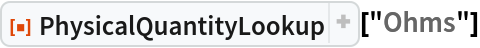Out=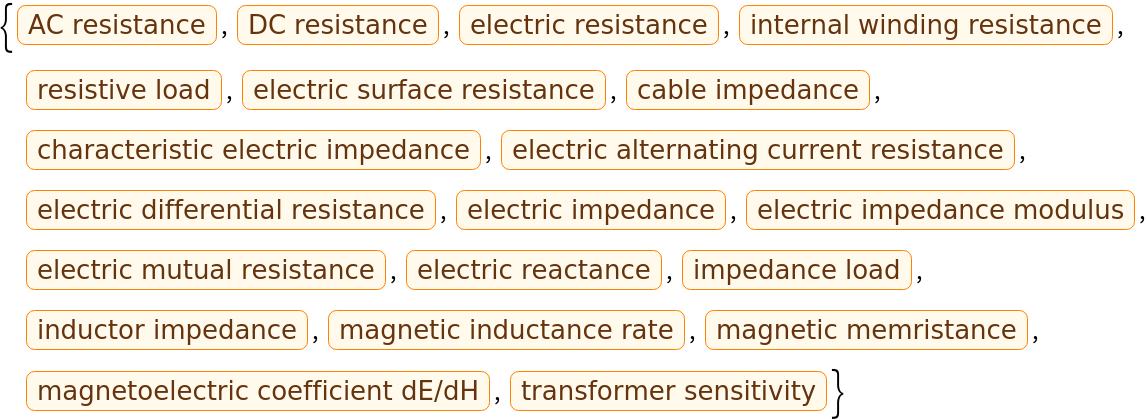Look for physical quantities that cover a quantity:

 In:=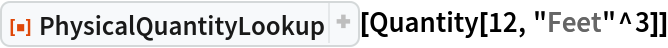Out=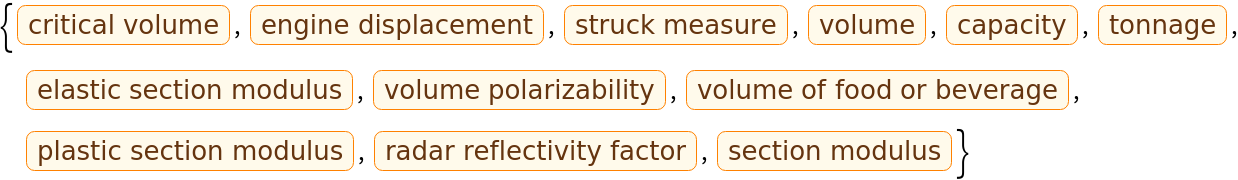Search by specifying unit dimensions:

 In:=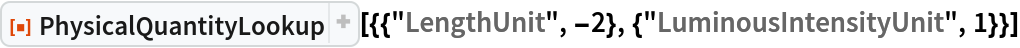Out=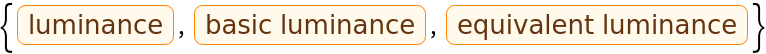### Scope (2)

Find the physical quantity corresponding to the gravitational constant:

 In:=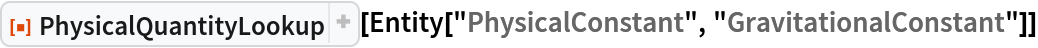Out=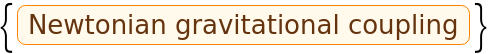Obtain the quantity variables for the physical quantities where available:

 In:=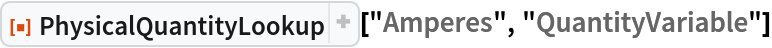Out=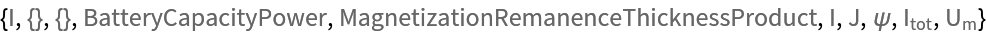Get the names for a given quantity unit:

 In:=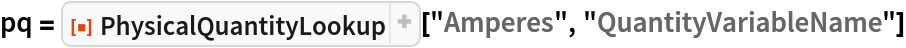Out=Construct custom variables for these names:

 In:=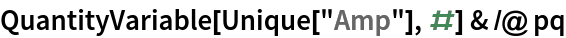Out=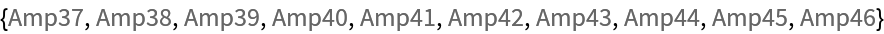### Applications (3)

Obtain the dimensions of energy:

 In:=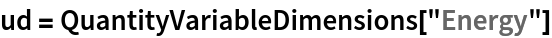Out=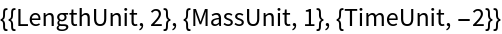Examine all physical quantities with these dimensions:

 In:=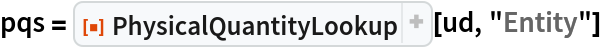Out=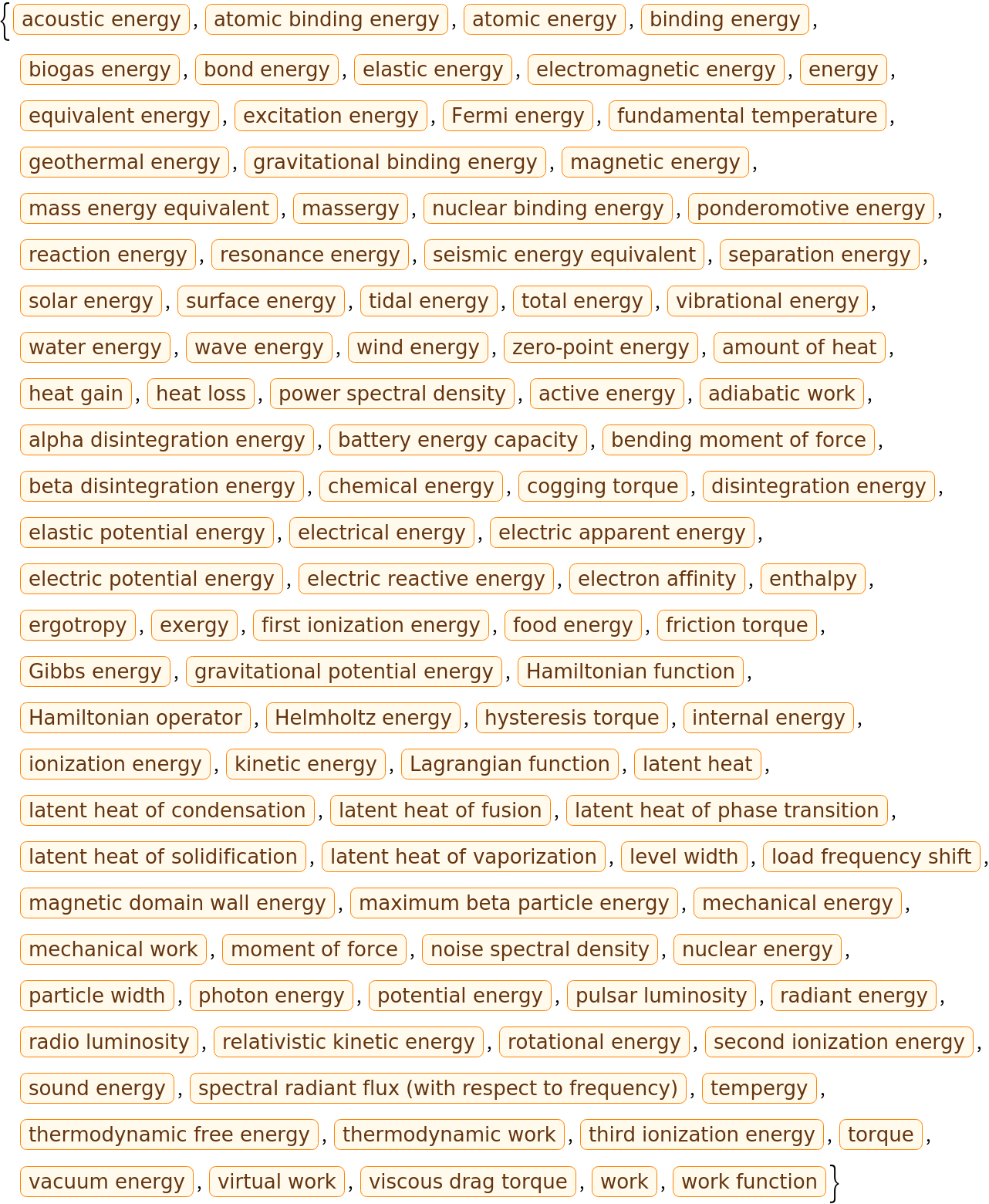Graph and group the physical quantities according to their base physical quantity:

 In:=Out=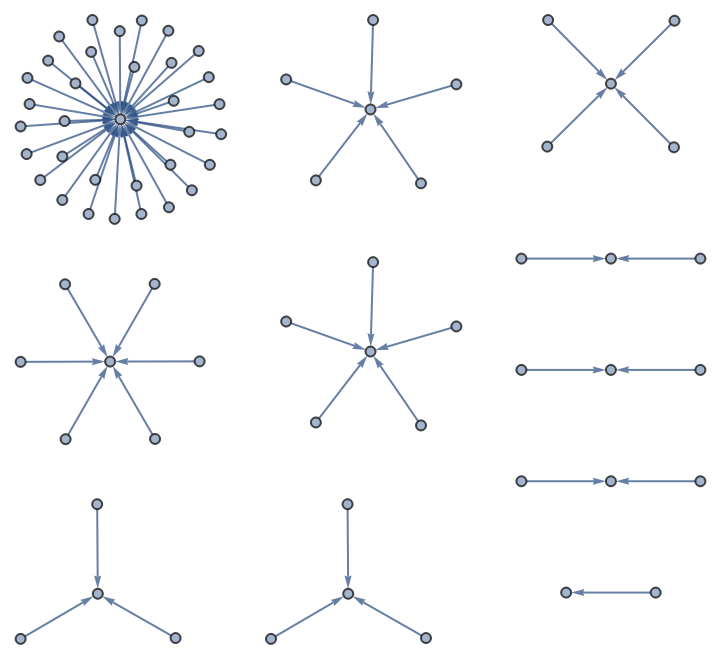### Properties and Relations (2)

Canonical units for physical quantities may differ from the unit used to find them:

 In:=Out=In:=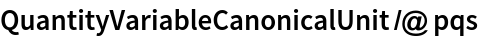Out=Unit dimensions can be determined with UnitDimensions or QuantityVariableDimensions:

 In:=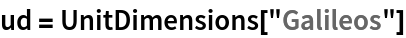Out=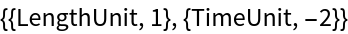In:=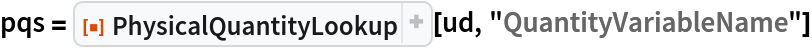Out=In:=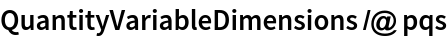Out=### Possible Issues (2)

Combinations of units can be queried by specifying them in a Quantity:

 In:=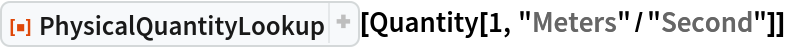Out=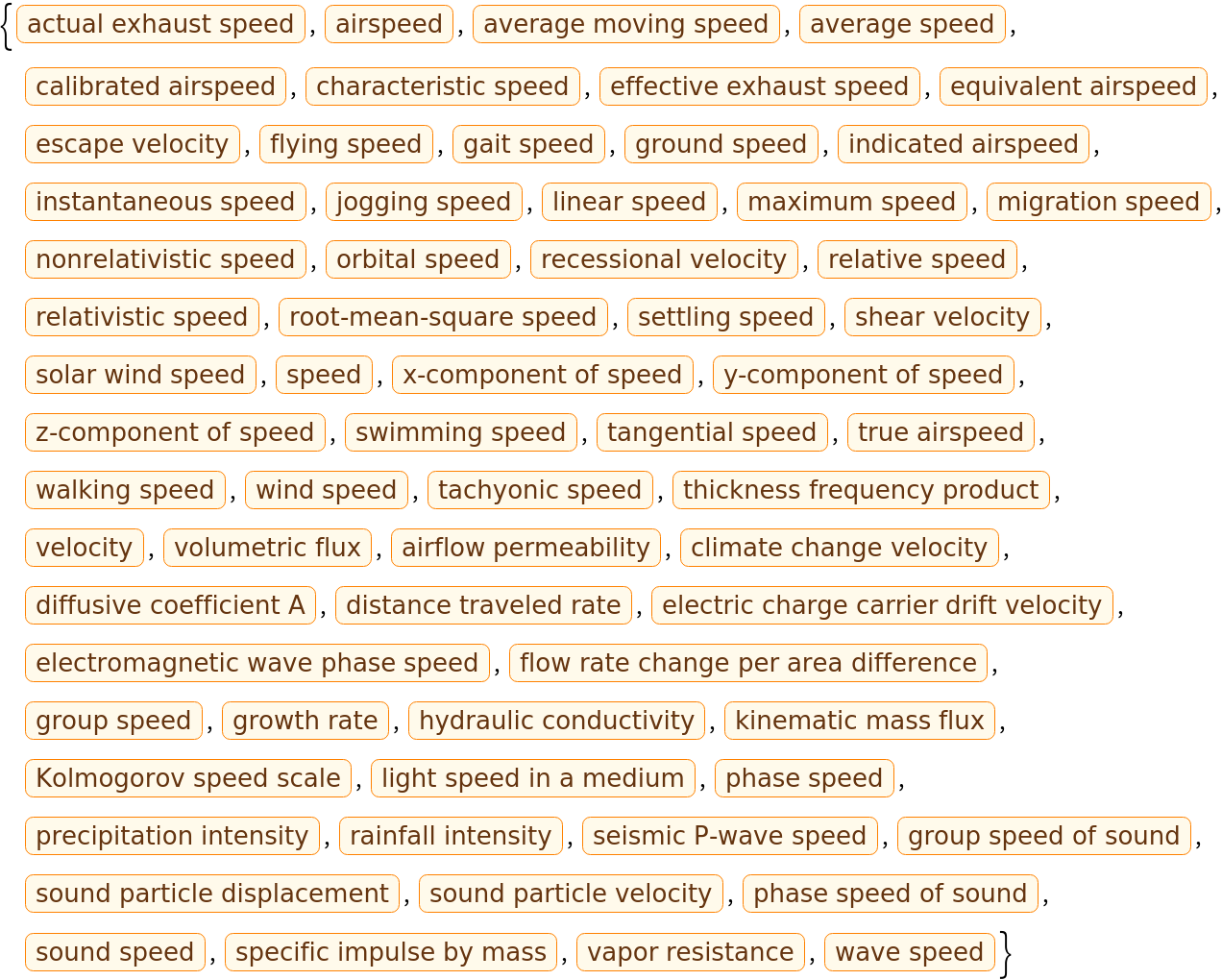Some combinations of units or unit dimensions do not have an associated physical quantity:

 In:=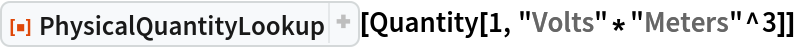Out=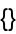Use the unit dimensions within a QuantityVariable to work with these sort of situations:

 In:=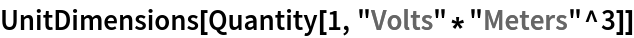Out=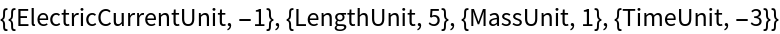In:=Out=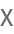In:=Out=In:=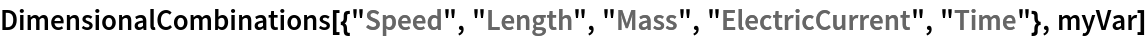Out=## Version History

• 1.0.0 – 28 March 2022

## Author Notes

PhysicalQuantityLookup uses entity class lookups and so relies on an internet connection.